## Solution to 1986 Problem 73

 We make the approximation that exactly two light beams of equal intensity reach the observer. One beam is reflected from the coating - air interface, and the other beam is reflected from the coating - glass interface. We also ignore any reflections except at these two interfaces. The index of refraction of the coating is greater than that of air, and the index of refraction of glass is greater than that of the coating, therefore, both beams will undergo a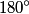$180^{\circ}$ phase shift when they are reflected. Therefore, the phase difference between the two beams is given by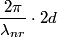\begin{align*}\frac{2 \pi}{\lambda_{nr}} \cdot 2 d\end{align*}where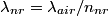$\lambda_{nr} = \lambda_{air}/n_{nr}$ is the wavelength of the light in the coating and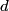$d$ is the thickness of the non-reflecting layer. We are actually given the wavelength of the light in the coating, i.e.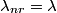$\lambda_{nr} = \lambda$. In order for destructive interference to occur, the phase difference must equal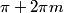$\pi + 2 \pi m$ where$m$ is an integer. Thus,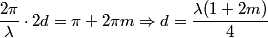\begin{align*}\frac{2 \pi}{\lambda} \cdot 2 d = \pi + 2 \pi m \Rightarrow d = \frac{\lambda (1 + 2 m)}{4}\end{align*}If we want to have the layer be as thin as possible, we should choose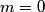$m = 0$. This then gives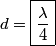\begin{align*}d = \boxed{\frac{\lambda}{4}}\end{align*}Therefore, answer (A) is correct.